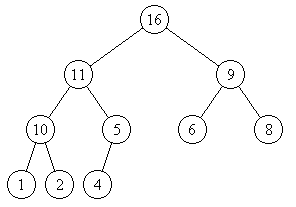Tuesday, December 30, 2008

Priority Queue & Heapsort

A priority queue is a queue where each item has a priority associated with it. And the item with the highest priority is at the top of the queue. So, you will be removing the highest priority items from the queue first.

A priority queue can be implemented using a heap where the heap is implemented using a complete binary tree. A complete binary tree is supposed to have the heap property if the root has higher priority than its child and each child also follows the same heap property.

Now, lets try to understand this thing in english.A complete binary tree is a tree filled from left to right. So, this is a complete binary tree. Also, it could be seen that for each node the root is always bigger than the child nodes. That is the heap property. And since the root has the highest priority and it is the first element, this is also a priority queue. If you remove the root, you will have to re-arrange the elements so that the new root again has the highest priority.

The benefit of using a heap structure is that inserting new element and removing root are handled in a constant O(log n) time, that is the time taken to re-arrange the elements. When the highest priority element is removed, we put the last element in the tree (lowest right element) at the root position. This new root is compared with both its children and if it has low priority as compared to either of its children, it is exchanged with the child. This goes on till the node resides at a place where its children are of lower priority and its parent is of higher priority. While inserting a new element, we place it at the last available node (remember, the tree should always be a complete tree), and move it up (basically follow the reverse procedure of removing root).

A heap of n elements could be easily stored using n sequential locations in an array. The left child node of node at position k is placed at position 2k, and the right child node is placed at 2k+1.

So, for the above heap, the elements would be stored as

16, 11, 9, 10, 5, 6, 8, 1, 2, 4

Lets check out the pseudo code for pushing and popping elements from the heap:

function heapPop(arr, count)
{
start = (count-2)/2;
while(start >= 0)
{
shiftDown(arr, start, count-1);
start = start=1;
}
}

function shiftDown(arr, start, end)
{
root = start;
while root*2+1 <= end // while root has only 1 child
{
child = root*2+1;
if ( child+1 < end ) and ( arr[child]<a[child+1] )
child = child+1;
if ( arr[root] < arr[child] )
{
swap(arr[root], arr[child]);
root = child;
}
else
return;
}
}

function heapPush( arr, count)
{
end = 1;
while (end < count )
{
shiftUp(arr, 0, end);
end = end+1;
}
}

function shiftUp(arr, start, end)
{
child = end;
while (child > start )
{
parent = floor((child-1)/2);
if(arr[parent] < arr[child])
{
swap(arr[parent],arr[child]);
child = parent;
}
else
return;
}
}

function heapSort(arr, count) // input is unsorted array "arr" of "count" elements
{
heapPop(arr, count);
end = count-1;
while(end > 0)
{
swap(arr[end],arr);
end = end-1;
heapPop(arr, count);
}
}